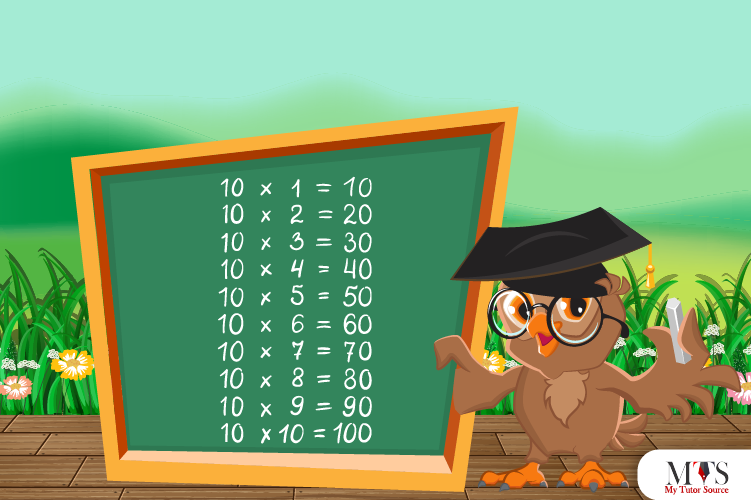# Multiplication Table of 10 – Tips to Memorize 10 Times Table & Example QuestionsHere comes the multiplication table of 10 – students’ favorite table so far! Teachers and math tutors encourage students to read and memorize 10 times table as they help solve and understand the number system and mathematical problems. Whether solving division, multiplication, LCM, HCF, or any other mathematical problem, a 10 times table will always be used.

The product of the multiplication table of 10 is a set of natural numbers and easy to memorize. This article has discussed everything related to the 10 times table, which helps you perform calculations and learn quickly.

## Table of 10

Below is the table of the whole number 10 up to 20. Give it a read.

10 x 1 = 10
10 x 2 = 20
10 x 3 = 30
10 x 4 = 40
10 x 5 = 50
10 x 6 = 60
10 x 7 = 70
10 x 8 = 80
10 x 9 = 90
10 x 10 = 100
10 x 11 = 110
10 x 12 = 120
10 x 13 = 130
10 x 14 = 140
10 x 15 = 150
10 x 16 = 160
10 x 17 = 170
10 x 18 = 180
10 x 19 = 190
10 x 20 = 200

## Method to Read Table of 10

Nobody can deny the importance of a 10 times table for performing mathematical calculations. All students must memorize it at their fingertips. To learn, one must know how to read properly, and, in this section, we have provided the table of 10 in words.

Ten ones are ten (10)
Ten twos are twenty (20)
Ten threes are thirty (30)
Ten fours are forty (40)
Ten fives are fifty (50)
Ten sixes are sixty (60)
Ten sevenths are seventy (70)
Ten eighths are eighty (80)
Ten nines are ninety (90)
Ten tens are hundred (100)
Ten elevens are one hundred and ten (110)
Ten twelves are one hundred and twenty (120)
Ten thirteenths are one hundred and thirty (130)
Ten fourteens are one hundred and forty (140)
Ten fifteens are one hundred and fifty (150)
Ten sixteenths are one hundred and sixty (160)
Ten seventeens are one hundred and seventy (170)
Ten eighteenths are one hundred and eighty (180)
Ten nineteens are one hundred and ninety (190)
Ten twenties are two hundred (200)

## Tips for Memorizing Multiplication Table of 10

Memorizing the 10 times table has many advantages. Among multiplication tables from 1 to 20, the table of 10 is the simplest and easiest to memorize. Follow the tips given below to remember it:

• The unit place of the product of the 10 times table is always zero, 0.
• To find the product of the next multiplier, skip the count of 10.
• The pattern of the 10 times table is simple. it is followed by natural numbers followed by 0
• Putting 0 next to the number of multiples of 10 will get the product of 10 times table. For example, to find the 8 x 10, write 0 next to 8. i.e., 80.

### Example Question of Table of 10

#### Question: Jia bought 10 chocolates, and each cost \$40. How much did she spend?

Solution:

Given that,
Number of chocolates: 10
Cost per chocolate: \$40
Therefore,
Total cost = 10 x 40 = 400
Hence, Jia spent \$400 total.

#### Question: Evaluate 10 times 9 minus 10 times 6?

Solution:

Given that,
10 times 9 minus 10 times 6
In mathematical form,
= 10 x 9 – 10 x 6
= 90 – 60
= 30
Hence, 10 times 9 minus 10 times six is 30.

#### Question: write pattern of 10 times table up to 15?

10, 20, 30, 40, 50, 60, 70, 80, 90, 100, 110, 120, 130, 140 and 150.

#### Question: Find the values of 10 times 7 plus 9 times 5. (Use the table of 10 and 9)

Solution:

Given that,
10 times 7 plus 9 times 5
In mathematical form,
= 10 x 7 – 9 x 5
= 70 – 45
= 25
Hence, the value of 10 times 7 plus 9 times 5 is 25.

#### Question: What is the value of 10 x 9 + 4 using the table of 10?

Solution:

Given that,
= 10 x 9 + 4
Using 10 times table
= 90 + 4
= 94
Hence, the value of 10 x 9 + 4 is 94

### Find Top Tutors in Your AreaWith over 3 years of experience in teaching, Chloe is very deeply connected with the topics that talk about the educational and general aspects of a student's life. Her writing has been very helpful for students to gain a better understanding of their academics and personal well-being. I’m also open to any suggestions that you might have! Please reach out to me at chloedaniel402 [at] gmail.com